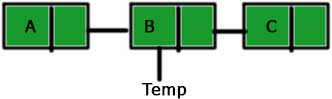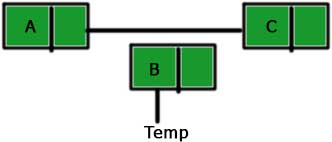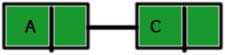# C++: Deletion of a given node from a linked list in C++

May 30, 2017 128694

### Previous:

Make sure that you are familiar with the concepts explained in the post(s) mentioned above before proceeding further.

We will proceed further by taking the linked list we made in the previous post.

#include <iostream>

using namespace std;

struct node
{
int data;
node *next;
};

{
private:
public:
{
tail = NULL;
}

{
node *tmp = new node;
tmp->data = n;
tmp->next = NULL;

{
tail = tmp;
}
else
{
tail->next = tmp;
tail = tail->next;
}
}

{
}

{
{
cout << "NULL" << endl;
}
else
{
}
}

static void concatenate(node *a,node *b)
{
if( a != NULL && b!= NULL )
{
if (a->next == NULL)
a->next = b;
else
concatenate(a->next,b);
}
else
{
cout << "Either a or b is NULL\n";
}
}

void front(int n)
{
node *tmp = new node;
tmp -> data = n;
}

void after(node *a, int value)
{
node* p = new node;
p->data = value;
p->next = a->next;
a->next = p;
}
};

int main()
{
a.front(3);
return 0;
}


We delete any node of a linked list by connecting the predecessor node of the node to be deleted by the successor node of the same node. For example, if we have a linked list a → b → c, then to delete the node ‘b’, we will connect ‘a’ to ‘c’ i.e., a → c. But this will make the node ‘b’ inaccessible and this type of inaccessible nodes are called garbage and we need to clean this garbage. We do this cleaning by the use of ‘delete’ operator. If you are not familiar with the ‘delete’ operator then you can visit the ‘Dynamic memory’ chapter of the C++ course. So, the steps to be followed for deletion of the node ‘B’ from the linked list A → B → C are as follows:

1. Create a temporary pointer to the node ‘B’.2. Connect node ‘A’ to ‘C’.3. Delete the node ‘B’.The code representing the above steps is:

void del (node *before_del)
{
node* temp;
temp = before_del->next;
before_del->next = temp->next;
delete temp;
}


Here, ‘before_node’ is the predecessor of the node to be deleted.
temp = before_del->next – We are making a temporary pointer to the node to be deleted.
before_del->next = temp->next – Connecting the predecessor of the node to be deleted with the successor of the node to be deleted.
delete temp – Making the ‘temp’ free.

And the overall code is:

#include <iostream>

using namespace std;

struct node
{
int data;
node *next;
};

{
private:
public:
{
tail = NULL;
}

{
node *tmp = new node;
tmp->data = n;
tmp->next = NULL;

{
tail = tmp;
}
else
{
tail->next = tmp;
tail = tail->next;
}
}

{
}

{
{
cout << "NULL" << endl;
}
else
{
}
}

static void concatenate(node *a,node *b)
{
if( a != NULL && b!= NULL )
{
if (a->next == NULL)
a->next = b;
else
concatenate(a->next,b);
}
else
{
cout << "Either a or b is NULL\n";
}
}

void front(int n)
{
node *tmp = new node;
tmp -> data = n;
}

void after(node *a, int value)
{
node* p = new node;
p->data = value;
p->next = a->next;
a->next = p;
}

void del (node *before_del)
{
node* temp;
temp = before_del->next;
before_del->next = temp->next;
delete temp;
}
};

int main()
{
a.front(3);